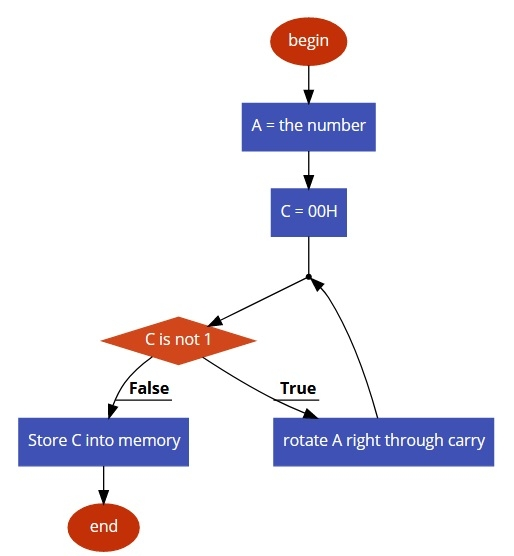# 8085 program to find the set bit of accumulator

Here we will see how to find the place of the set bit of the Accumulator data.

## Problem Statement

Write 8085 Assembly language program to find the position where the bit is 1. In the accumulator all bits are 0, but only one bit is 1. We have to get the position of the bit which is 1. The position will be displayed in decimal from 1 to 8.

## Discussion

We are taking the number like (0010 0000). The place value is 6. So we are rotating the number to the right through carry. If the carry bit is 1, then we break the loop and get the result at memory location F051.

Data
F050
20

Data
F050
80

## Flow Diagram## Program

HEX Codes
Labels
Mnemonics
F000
3A, 50 F0

LDA F050
Take the number into accumulator into memory
F003
0E, 00

MVI C,00H
Clear C flag
F005
1F
LOOP
RAR
Rotate Acc right with carry
F006
0C

INR C
increase C by 1
F007
D2, 05, F0

JNC LOOP
F00A
79

MOV A,C
Take value from C to A
F00B
32, 51, 50

STA F051
Store result into memory
F00E
76

HLT
Terminate the program

Data
F051
06

Data
F150
08